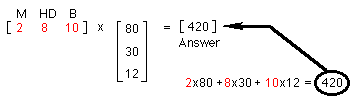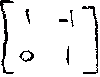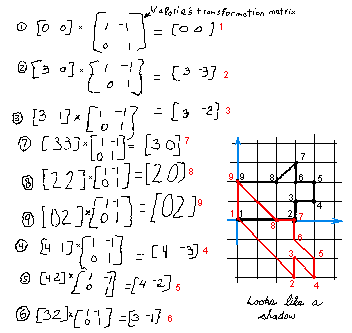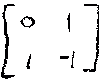## Sample Problems from "Changing Shapes With Matrices"

Ch. 1: Plotting points - graphing linear equations -See chapter 6 in "Calculus By and For Young People- Worksheets"

Ch. 2: Grocery-store arithmetic to multiply matrices
We go to the store to buy some things. What shall we buy? OK, we'll buy some milk(M), some hotdogs(HD) and some buns(B). How much of each? 2 cartons of milk, 8 hot dogs and 10 buns.  The milk costs 80'/carton, the hot dogs 30' each, and the buns cost 12' each. We write this information in matrix form below. How much does everything cost?Everything cost 420' or \$4.20. One multiplies by going across to the right on the first matrix and down the second matrix, multiplying then adding.

Ch. 3: Steps to do a transformation and Valorie's work
Steps to do a geometric transformation using matrices:
1. Make a shape. The shape I chose was the "dog" (simple, not too many points).
2. Pick out about 10 points on the shape.
3. Write down the coordinates of these points.
4. Number each of these points to make it easier to keep track of things.
5. Pick a 2x2 matrix using only 1's or 0's or -1's (for now). This will be your transformation matrix. For example, Valorie chose the matrix:6. Multiply each point (as a matrix) by your transformation matrix to get a new point.
7. Plot each new point and number it, keeping a correspondence with the old points.
8. Connect the new points as you go, using a color different from the original shape.
9. Complete all the new points and close the shape (if it's a closed figure), then look at the new shape carefully. Try to figure out what your matrix did to the original shape. Then ask questions about the situation.Ch. 4 Asking questions & Other Student Work
Try changing the doggie with Mark's matrix:What matrix would rotate the dog 90 degrees counterclockwise?
What matrix would reflect the dog in the x-axis?
How many 2x2 matrices with 0's, 1's, and -1's can you make?